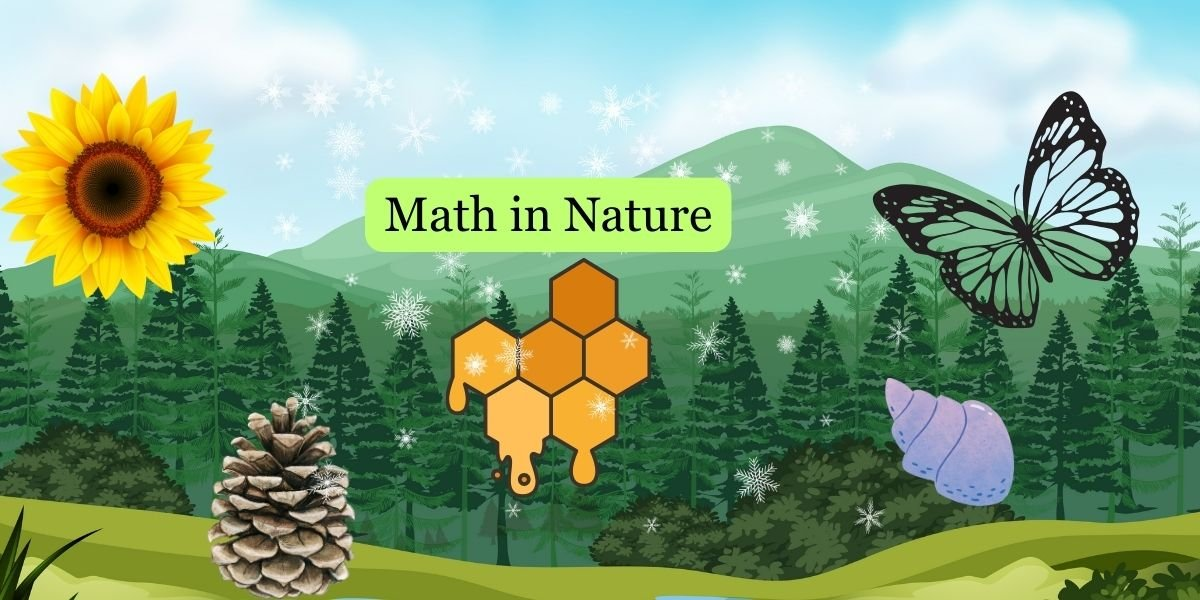Mathematics is not just an abstract concept confined to classrooms; it’s deeply embedded in the patterns and structures of the natural world. Here are some examples of math in nature:

1. Fibonacci Sequence in Sunflowers:
• The arrangement of seeds in a sunflower follows the Fibonacci sequence. Each spiral in the sunflower seed head represents a Fibonacci number, showcasing the mathematical elegance in nature.
2. Symmetry in Butterflies:
• The wings of butterflies often exhibit symmetry. The patterns and colors on one side mirror those on the other, demonstrating mathematical principles of symmetry.
3. Golden Ratio in Pinecones:
• Pinecones and pineapples often display the golden ratio, a mathematical constant that appears in various natural structures. The ratio of the length of successive segments is approximately equal to 1.618.
4. Fractals in Trees and Leaves:
• Trees and leaves exhibit fractal patterns. The branching of trees and the veins in leaves repeat smaller versions of the overall shape, creating self-repeating patterns.
5. Hexagonal Cells in Honeycombs:
• Honeybees construct their hives using hexagonal cells. The hexagon is the most efficient shape for packing cells, allowing bees to maximize space and use less wax.
6. Spirals in Shells:
• Many seashells, such as snail shells, display logarithmic spirals. These spirals follow mathematical rules and are often associated with the Fibonacci sequence.
7. Hexagonal Symmetry in Snowflakes:
• Snowflakes, with their intricate patterns, often exhibit hexagonal symmetry. The unique shapes of snowflakes arise from the molecular structure of ice and the conditions under which they form.
8. Spirals in Pinecones:
• Pinecones often showcase spirals that follow the Fibonacci sequence. The arrangement of scales on a pinecone reflects the mathematical patterns found in nature.
9. Geometric Patterns in Beehives:
• Beehives are constructed with hexagonal cells, providing a strong and efficient structure. The bees use their instinctive understanding of geometry to create a hive that meets their needs.
10. Geodesic Domes in Some Plants:
• Some plants, like the Buckminster fullerene (buckyball plant), display structures reminiscent of geodesic domes. These geometric patterns provide strength and efficiency in resource use.

These examples illustrate that mathematics is an intrinsic part of the natural world, influencing the growth, structure, and patterns observed in various living organisms and natural formations. Exploring these mathematical connections enhances our appreciation for the beauty and order in nature.